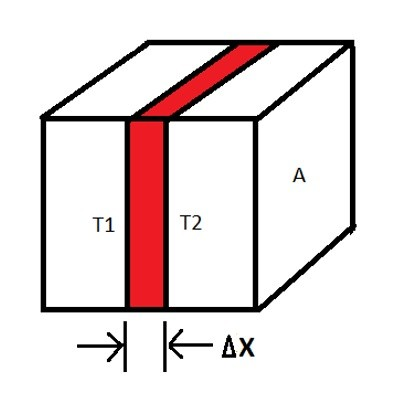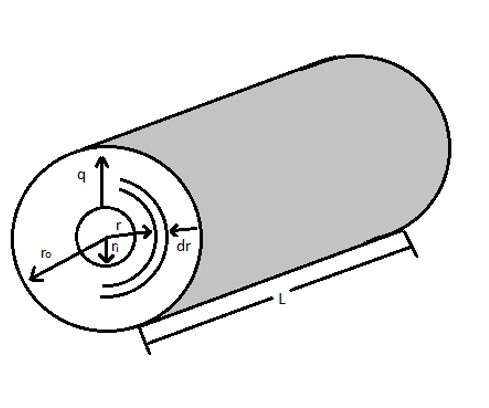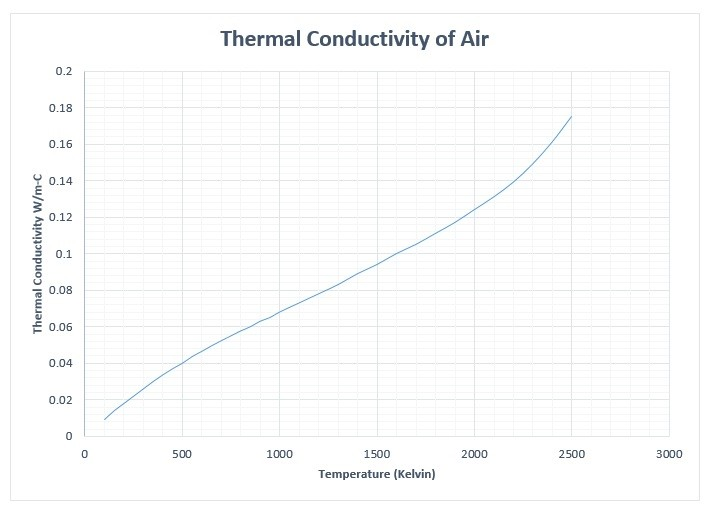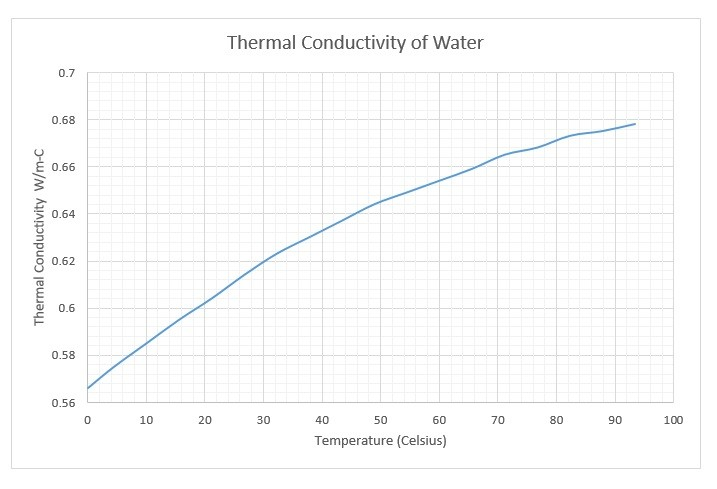# Conduction

Heat transfer is caused when there is a temperature gradient that exist within the body of an object. The transfer of heat occurs from the high temperature region to the lower temperature region. Conduction is the rate of heat transfer through a body or through multiple bodies that are in direct contact with each other. In order to calculate the rate of heat transfer the following equation would be used.

(eq 1)  $q_x = -kA\frac{ΔT}{Δx}$

In the equation above k represents the thermal conductivity of the material of the object of interest. A is the cross-sectional area. T/x is the temperature change in the direction of the heat flow. There is a negative sign in the equation to satisfy the second law of thermodynamics. The image below shows conductive heat transfer in one direction.There are also certain cases where you might want to find the thermal resistance through material for a specified distance. Refer to the equation below.

(eq 2)  $R=\frac{Δx}{kA}$

## Example

What would be the rate of heat transfer for the following problem? A plate that is 0.1m thick has one side of the plate maintained at 300 degrees C. The other side is maintained at 75 degrees C. What is the rate of heat transfer through the plate? The thermal conductivity of the material is 370 W/ (m-C).

The first step is to rearrange equation 1 so that the problem can be solved.

(eq 3) $\frac{q}{A}=-k\frac{ΔT}{Δx}$

Now the rate of heat transfer can be determined.

(eq 4) $\frac{q}{A} = -370\left(\frac{75-300}{0.1}\right) = 832.5 \frac{kW}{m^2}$

## Conduction Through Cylinders

Currently I have only talked about cube type shapes. However, there is also times when there could be conduction through a radial object such as a cylinder. Refer to the image below.For a cylinder equation 1 would be modified to the following.

(eq 5) $q_r = -kA_r \frac{dT}{dr}$

(eq 6) $A_r = 2πL$

Finally, to calculate the resistance to heat transfer in a cylinder the following equation would be used.

(eq 7) $R = \frac{ln\left(r_o/r_i\right)}{2πkL}$

## Thermal Conductivity Coefficient of Different Materials

Below is a table showing the thermal Conductivity of Common Materials at zero degrees C. Be aware that thermal conductivity is temperature dependent.

 Material Thermal Conductivity (W/m-C) Copper (pure) 385 Aluminum (pure) 202 Chrome-Nickel Steel (18% Cr, 8% Ni) 16.3 Hard Rubber 0.15 Water 0.556 Air 0.024

Below is a graph showing the thermal conductivity of air in respect to temperature.Below is a graph showing the thermal conductivity of water in respect to temperature.Below is a graph showing the thermal conductivity of various metals in respect to temperature.